# ISEE Upper Level Math : How to find mean

## Example Questions

← Previous 1 3 4

### Example Question #1 : How To Find Mean

Consider the following set of scores from a math test. What is the mean of these scores?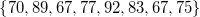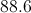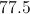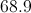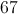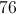Explanation:

To find the mean, first sum up all the values.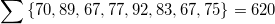The divide the result by the number of values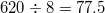### Example Question #2 : How To Find Mean

The mean of six numbers is 77. What is their sum?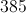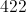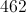It cannot be determined from the information given.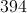Explanation:

The mean of six numbers is their sum divided by 6, so the sum is the mean multiplied by 6. This is: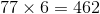### Example Question #3 : How To Find Mean

Sally's numeric grade in her economics class is determined by four equally weighted hourly tests, a midterm weighted twice as much as an hourly test, and a final weighted three times as much as an hourly test. The highest score possible on each is 100.

Going into finals week, Sally's hourly test scores are 89, 85, 84, and 87, and her midterm score is 93. What must Sally make on her final at minimum in order to average 90 or more for the term?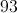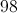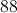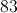It is impossible for Sally to achieve this average this term.Explanation:

Sally's grade is a weighted mean in which her hourly tests have weight 1, her midterm has weight 2, and her final has weight 3. If we callher score on the final, then her course score will be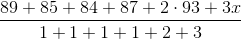,

which simplifies to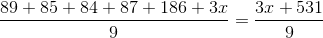.

Since Sally wants at least a 90 average for the term, we can set up and solve the inequality: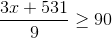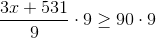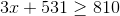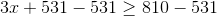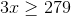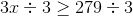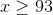Sally must score at least 93 on the final.

### Example Question #1 : How To Find Mean

Fred's course average in French class is the average of the best five of his six hourly test scores. Going into finals week, Fred has scores of 78, 77, 84, 89, and 72. How much, at minimum, must Fred score on his sixth test in order to make an average of 80 or better for the term?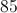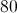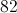Fred is already assured an average of 80 or better for the term.

Fred is already assured an average of 80 or better for the term.

Explanation:

If Fred does not take the sixth test or gets a 0 on it, he will receive the average of his first five tests. This is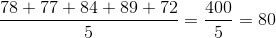.

Since he can only improve his class grade by taking the sixth test, Fred is already assured of an average of 80 or better.

### Example Question #1 : Mean

Alice's numeric grade in her physics class is determined by four equally weighted hourly tests and a final, weighted twice as much as an hourly test. The highest score possible on each is 100.

Going into finals week, Alice's hourly test scores are 76, 85, 82, and 87. What must Alice make on her final, at minimum, in order to average 90 or more for the term?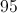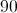It is impossible for Alice to achieve an average of 90 or better for the term.

It is impossible for Alice to achieve an average of 90 or better for the term.

Explanation:

Alice's grade is a weighted mean in which her hourly tests have weight 1 and her final has weight 2. If we callher final, then her term score will be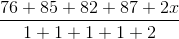,

which simplifies to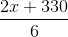.

Since she wants her score to be 90 or better, we solve this inequality: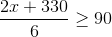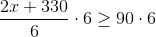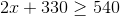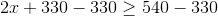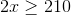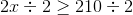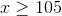Since it is established that 100 is the maximum score, Alice cannot achieve an average of 90 or more for the term.

### Example Question #6 : How To Find Mean

Eric has taken four tests in his English class. His scores on the tests are 94, 87, 95, and 89. The upcoming final exam will be weighted as much as each of the previous four tests. What is the lowest score Eric can get on the final exam and still have a mean score of no less than 90?

86

85

84

87

85

Explanation:

The first step here, since you're working with the average (or mean), is to determine what is the sum of five test scores that will result in Eric earning at least a mean score of 90.

Start with the fact that he will need to make at least a 90 for his average score. Since you already have the average score, you're working backwards. You need to multiply 90 by 5 for the 5 test scores that will be used to get that average: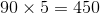This tells you that the five scores - the four test scores and the final exam - will need to add up to at least 450 in total.

You know the four original test scores, so you can add those up: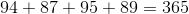So you know the four test scores add up to 365. To get an average of at least 90, the fifth score must bring that sum to at least 450. To find the minimum final exam test score, you subtract 365 from 450: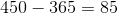This tells you that Eric must score at least an 85 on the final exam to achieve an overall mean score of 90.

### Example Question #7 : How To Find Mean

Consider the time Becky spends doing homework each night. What is the average number of hours Becky spends on homework each night (consider only Monday-Thursday)?

Monday: 3.2 hours

Tuesday: 1.1 hours

Wednesday: 2.7 hours

Thursday: 0.9 hours

1.925 hours

1.900 hours

1.950 hours

1.975 hours

1.975 hours

Explanation:

To calculate the average, find the sum of the total hours divided by the number of nights: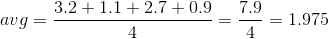### Example Question #8 : How To Find Mean

The mean of the following numbers is. Solve for.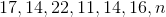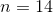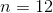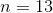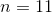Explanation:

The mean is determined by dividing the sum of the values by the total number of values: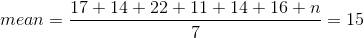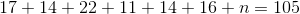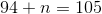### Example Question #9 : How To Find Mean

What is the mean of the first ten prime numbers?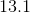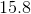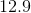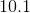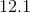Explanation:

The first ten primes form the data set: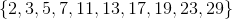Add them, and divide by: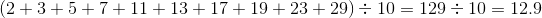### Example Question #10 : How To Find Mean

Consider the following set of scores from a math test. If the mean is, give.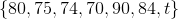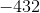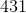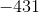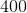Explanation:

Mean of the scores can be calculated as: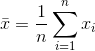Where: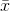is the mean of a data set,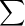indicates the sum of the data values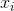,  andis the number of data values. So we can write: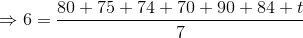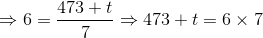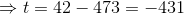← Previous 1 3 4